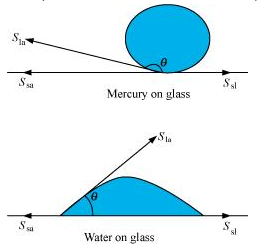## Pages

### Mechanical Properties of Fluids NCERT Solutions Class 11 Physics - Solved Exercise Question 10.2

Question 10.2:
Explain why
(a) The angle of contact of mercury with glass is obtuse, while that of water with glass is acute.
(b) Water on a clean glass surface tends to spread out while mercury on the same surface tends to form drops. (Put differently, water wets glass while mercury does not.)
(c) Surface tension of a liquid is independent of the area of the surface
(d) Water with detergent dissolved in it should have small angles of contact.
(e) A drop of liquid under no external forces is always spherical in shape
Solution:
(a) The angle between the tangent to the liquid surface at the point of contact and the surface inside the liquid is called the angle of contact (θ), as shown in the given figure.Sla, Ssa, and Ssl are the respective interfacial tensions between the liquid-air, solid-air, and solid-liquid interfaces. At the line of contact, the surface forces between the three media must be in equilibrium, i.e.,
Cosθ = (Ssa - Sla) / Sla
The angle of contact θ , is obtuse if Ssa < Sla (as in the case of mercury on glass). This angle is acute if Ssl < Sla (as in the case of water on glass).

(b) Mercury molecules (which make an obtuse angle with glass) have a strong force of attraction between themselves and a weak force of attraction toward solids. Hence, they tend to form drops.
On the other hand, water molecules make acute angles with glass. They have a weak force of attraction between themselves and a strong force of attraction toward solids. Hence, they tend to spread out.

(c) Surface tension is the force acting per unit length at the interface between the plane of a liquid and any other surface. This force is independent of the area of the liquid surface. Hence, surface tension is also independent of the area of the liquid surface.

(d) Water with detergent dissolved in it has small angles of contact (θ). This is because for a small θ, there is a fast capillary rise of the detergent in the cloth. The capillary rise of a liquid is directly proportional to the cosine of the angle of contact (θ). If θ is small, then cosθ will be large and the rise of the detergent water in the cloth will be fast.

(e) A liquid tends to acquire the minimum surface area because of the presence of surface tension. The surface area of a sphere is the minimum for a given volume. Hence, under no external forces, liquid drops always take spherical shape.